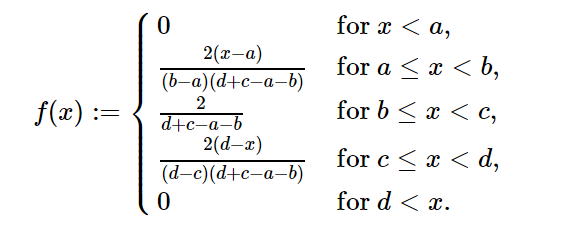Related Articles

# sympy.stats.Trapezoidal() in Python

• Last Updated : 08 Jun, 2020

With the help of `sympy.stats.Trapezoidal()` method, we can get the continuous random variable which represents the Trapezoidal distribution by using this method.Syntax : `sympy.stats.Trapezoidal(name, a, b, c, d)`
Where, a, b, c and d are real number.
Return : Return the continuous random variable.

Example #1 :
In this example we can see that by using `sympy.stats.Trapezoidal()` method, we are able to get the continuous random variable representing Trapezoidal distribution by using this method.

 `# Import sympy and Trapezoidal``from` `sympy.stats ``import` `Trapezoidal, density``from` `sympy ``import` `Symbol, pprint`` ` `z ``=` `Symbol(``"z"``)``a ``=` `Symbol(``"a"``, positive ``=` `True``)``b ``=` `Symbol(``"b"``, positive ``=` `True``)``c ``=` `Symbol(``"c"``, positive ``=` `True``)``d ``=` `Symbol(``"d"``, positive ``=` `True``)`` ` `# Using sympy.stats.Trapezoidal() method``X ``=` `Trapezoidal(``"x"``, a, b, c, d)``gfg ``=` `density(X)(z)`` ` `pprint(gfg)`

Output :

/ -2*a + 2*z
|————————- for And(a z)
|(-a + b)*(-a – b + c + d)
|
| 2
| ————– for And(b z)
= z, c <= z)
|(-c + d)*(-a – b + c + d)
|
\ 0 otherwise

Example #2 :

 `# Import sympy and Trapezoidal``from` `sympy.stats ``import` `Trapezoidal, density``from` `sympy ``import` `Symbol, pprint`` ` `z ``=` `0.43``a ``=` `2``b ``=` `4``c ``=` `5``d ``=` `8`` ` `# Using sympy.stats.Trapezoidal() method``X ``=` `Trapezoidal(``"x"``, a, b, c, d)``gfg ``=` `density(X)(z)`` ` `pprint(gfg)`

Output :

0

Attention geek! Strengthen your foundations with the Python Programming Foundation Course and learn the basics.

To begin with, your interview preparations Enhance your Data Structures concepts with the Python DS Course. And to begin with your Machine Learning Journey, join the Machine Learning – Basic Level Course

My Personal Notes arrow_drop_up